# Test: Single Correct MCQs: Structure of Atom | JEE Advanced

## 37 Questions MCQ Test Chemistry 35 Years JEE Main & Advanced Past year Papers | Test: Single Correct MCQs: Structure of Atom | JEE Advanced

Description
Attempt Test: Single Correct MCQs: Structure of Atom | JEE Advanced | 37 questions in 70 minutes | Mock test for JEE preparation | Free important questions MCQ to study Chemistry 35 Years JEE Main & Advanced Past year Papers for JEE Exam | Download free PDF with solutions
QUESTION: 1

### The number of neutrons in dipositive zinc ion with mass number 70 is (1979)

Solution:

No. of neutrons = Mass number – Atomic number
= 70 – 30 = 40.

QUESTION: 2

### Rutherford’s experiment on scattering of a-particles showed for the first time that the atom has (1981 - 1 Mark)

Solution:

Rutherford’s scattering experiment led to the discovery of nucleus.

QUESTION: 3

### Any p-orbital can accommodate upto (1983 - 1 Mark)

Solution:

One p-orbital can accommodate up to two electrons with opposite spin while p-subshell can accommodate upto six electrons.

QUESTION: 4

The principal quantum number of an atom is related to the (1983 - 1 Mark)

Solution:

The principal quantum number (n) is related to the size of the orbital (n = 1, 2, 3.....)

QUESTION: 5

Rutherford’s scattering experiment is related to the size of the (1983 - 1 Mark)

Solution:

According to Rutherford's experiment. "The central part consisting of whole of the positive charge and most of the mass, called nucleus, is extremely small in size compared to the size of the atom."

QUESTION: 6

The increasing order (lowest first) for the values of e/m (charge/mass) for electron (e), proton (p), neutron (n) and alpha particle (α) is : (1984 - 1 Mark)

Solution: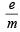for neutron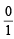= 0;  α-particle =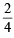= 0.5;

proton =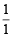= 1; electron =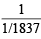= 1837

QUESTION: 7

Correct set of four quantum numbers for the valence (outermost) electron of rubidium (Z = 37) is : (1984 - 1 Mark)

Solution:

Rb has the configuration : 1s2 2s2p6 3s2p6d10 4s2p6 5s1; so n = 5, l = 0, m = 0 and s = +½ is correct set of quantum numbers for valence shell electron of Rb.

QUESTION: 8

Which electronic level would allow the hydrogen atom to absorb a photon but not to emit a photon? (1984 - 1 Mark)

Solution:

NOTE : Energy is emitted when electron falls from higher energy level to lower energy level and energy is absorbed when electron moves from lower level to higher level. 1s is the lowest energy level of electron in an atom.
∴ An electron in 1s level of hydrogen can absorb energy but cannot emit energy.

QUESTION: 9

Bohr model can explain : (1985 - 1 Mark)

Solution:

Bohr model can explain spectrum of atoms/ions containing one electron only.

QUESTION: 10

The radius of an atomic nucleus is of the order of : (1985 - 1 Mark)

Solution:

The radius of nucleus is of the order of 1.5 × 10–13 to 6.5 × 10–13 cm or 1.5 to 6.5 Fermi (1 Fermi = 10–13 cm)

QUESTION: 11

Electromagnetic radiation with maximum wavelength is : (1985 - 1 Mark)

Solution:

TIPS/Formulae : The following is the increasing order of wavelength or decreasing order of energy of electromagnetic radiations :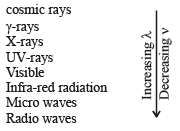Among given choices radiowaves have maximum wavelength.

QUESTION: 12

Rutherford’s alpha particle scattering experiment eventually led to the conclusion that : (1986 - 1 Mark)

Solution:

Electrons in an atom occupy the extra nuclear region.

QUESTION: 13

Which on e of th e following sets of quantum numbers represents an impossible arrangement? (1986 - 1 Mark)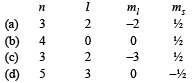Solution:

If l = 2, m ≠ –3, m will vary from –2 to +2. i.e. possible values of m are –2, –1, 0, + 1 and + 2.

QUESTION: 14

The ratio of the energy of a photon of 2000Å wavelength radiation to that of 4000Å radiation is : (1986 - 1 Mark)

Solution: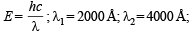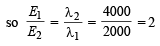QUESTION: 15

The triad of nuclei that is isotonic is (1988 - 1 Mark)

Solution:

Isotones have same number of neutrons. All atoms in triad (a) have same number of neutrons (= A – Z = 8).

QUESTION: 16

The wavelength of a spectral line for an electronic transition is inversely related to : (1988 - 1 Mark)

Solution:

Difference in the energy of the energy levels involved in the transition.

QUESTION: 17

The orbital diagram in which the Aufbau principle is violated is : (1988 - 1 Mark)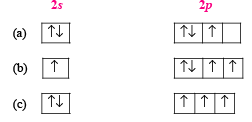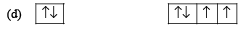Solution:

According to Aufbau principle, the orbital of lower energy (2s) should be fully filled before the filling of orbital of higher energy starts.

QUESTION: 18

The outermost electronic configuration of the most electronegative element is (1988 - 1 Mark)

Solution:

TIPS/Formulae : The element having highest tendency to accept the electron will be most electronegative element.
Configuration ns2, np5 means it requires only one electron to attain nearest noble gas configuration. So, it will be most electronegative element among given choices.

QUESTION: 19

The correct ground state electronic configuration of chromium atom is : (1989 - 1 Mark)

Solution:

NOTE : Exactly half filled orbitals are more stable than nearly half filled orbitals.
Cr (At. no. 24) has configuration [Ar] 3d5, 4s1.

QUESTION: 20

The correct set of quantum numbers for the unpaired electron of chlorine atom is : (1989 - 1 Mark)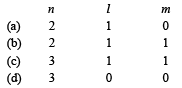Solution:

Electronic configuration of chlorine is [Ne] 3s2, 3p5
∴ Unpaired electron is found in 3p sub-shell.
∴ n = 3, l = 1, m = 1

QUESTION: 21

Which of the following does not characterise X-rays? (1992 - 1 Mark)

Solution:

X-rays can ionise gases and cannot get deflected by electric and magnetic fields, wavelength of these rays is 150 to 0.1Å. Thus the wavelength of X-rays is shorter than that of u.v. rays.

QUESTION: 22

Which of the following relates to photons both as wave motion and as a stream of particles? (1992 - 1 Mark)

Solution:

As packet of energy equal to hv; as wave having frequency v.

QUESTION: 23

A 3p orbital has : (1995S)

Solution:

TIPS/Formulae : Total nodes = n – l
No. of radial nodes = n – l – 1
No. of angular nodes = l
For 3p sub-shell, n = 3, l = 1
∴ No. of radial nodes = n – l – 1 = 3 – 1 – 1 = 1
∴ No. of angular nodes = l = 1

QUESTION: 24

The orbital angular momentum of an electron in 2s orbital is: (1996 - 1 Mark)

Solution:

TIPS/Formulae : Orbital angular momentum (mvr) =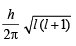For 2s orbital, l (azimuthal quantum number) = 0

∴ Orbital angular momentum = 0.

QUESTION: 25

For a d-electron, the orbital angular momentum is (1997 - 1 Mark)

Solution:

The expression for orbital angular momentum is Angular momentum =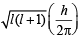For d orbital, l = 2.
Hence,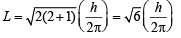QUESTION: 26

The electron s, iden tified by quantum numbers n and l, (i) n = 4, l = 1, (ii) n = 4, l = 0, (iii) n = 3, l = 2, and (iv) n = 3, l = 1 can be placed in order of increasing energy, from the lowest to highest, as (1999 - 2 Marks)

Solution:

TIPS/Formulae : The two guiding rules to arrange the various orbitals in the increasing energy are:
(i) Energy of an orbital increases with increase in the value of n + l.
(ii) Of orbitals having the same value of n + l, the orbital with lower value of n has lower energy.
Thus for the given orbitals, we have
(i)   n + l = 4 + 1 = 5
(ii)  n + l = 4 + 0 = 4
(iii) n + l = 3 + 2 = 5
(iv) n + l = 3 + 1 = 4
Hence, the order of increasing energy is (iv) < (ii) < (iii) < (i)

QUESTION: 27

The number of nodal planes in a px orbital is (2000S)

Solution:

px orbital being dumbell shaped, have number of nodal planes = 1, in yz plane.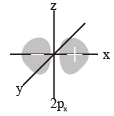QUESTION: 28

The electronic configuration of an element is 1s2, 2s2 2p6, 3s2 3p6 3d5, 4s1. This represents its (2000S)

Solution:

3d 54s1 system is more stable than 3d44s2, hence former is the ground state configuration.

QUESTION: 29

The wavelength associated with a golf ball weighing 200 g and moving at a speed of 5 m/h is of the order (2001S)

Solution:

TIPS/Formulae : According to de-Broglie’s equation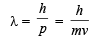Given, h = 6.6 × 10–34 Js, m = 200 × 10–3 kg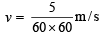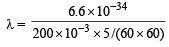= 2.38 × 10–30 m

QUESTION: 30

The quantum numbers +1/2 and –1/2 for the electron spin represent (2001S)

Solution:

The term spin implies that this magnetic moment is produced by the electron charge as the electron rotates about its own axis. Although this conveys a vivid mental picture of the source of the magnetism, the electron is not an extended body and its rotation is meaningless. Electron spin has no classical counterpart; the magnetic moment is a consequence of relativistic shifts in local space and time due to the high effective velocity of the electron in the atom.

QUESTION: 31

Rurtherford's experiment, which established the nuclear model of the atom, used a beam of (2002S)

Solution:

Rutherfor d's experiment was actually α -par ticle scattering experiment. α -Particle is doubly positively charged heliumion i.e., He - nucleus.

QUESTION: 32

If the nitrogen atom has electronic configuration 1s7, it would have energy lower than that of the normal ground state configuration 1s22s22p3, because the electrons would be closer to the nucleus. Yet 1s7 is not observed because it violates.(2002S)

Solution:

As per Pauli Exclusion Principle  "no two electrons in the same atom can have all the four quantum numbers equal or an orbital cannot contain more than two electrons and it can accommodate two electrons only when their directions of spins are opposite".

QUESTION: 33

The radius of which of the following orbit is same as that of the first Bohr ’s orbit of hydrogen atom? (2004S)

Solution:

TIPS/Formulae :​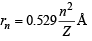For hydrogen, n = 1 and Z = 1 ; ∴ rH = 0.529

For Be3+, n = 2 and Z = 4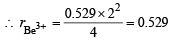QUESTION: 34

The n umber of radial nodes of 3s and 2p orbitals are respectively (2005S)

Solution:

TIPS/Formulae : Number of radial nodes = (n - l - 1)
For 3s: n = 3, l = 0 (Number of radial node = 2)
For 2p: n =2, l = 1 (Number of radial node = 0)

QUESTION: 35

Given that the abundances of isotopes 54Fe, 56Fe and 57 Fe are 5%, 90% and 5%, respectively, the atomic mass of Fe is

Solution:

Average atomic mass of Fe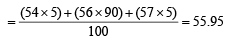QUESTION: 36

The kinetic energy of an electron in the second Bohr orbit of a hydrogen atom is [a0 is Bohr radius] : (2012)

Solution:

As per Bohr’s postulate,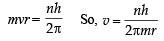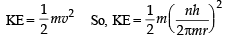Since,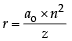So, for 2nd Bohr orbit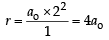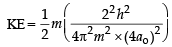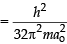QUESTION: 37

P is the probability of finding the 1s electron of hydrogen atom in a spherical shell of infinitesimal thickness, dr, at a distance r from the nucleus. The volume of this shell is 4πr2dr. The qualitative sketch of the dependence of P on r is (JEE Adv. 2016)

Solution:

Radial probability function curve for 1s is (D).
Here P is 4πr2R2.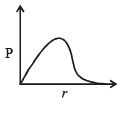Use Code STAYHOME200 and get INR 200 additional OFF Use Coupon Code